• ## matlabxlabel位置设置

万次阅读 2020-03-20 17:54:40
xlabel(‘time’,‘FontSize’,12); 如果没有设置位置，默认是在中间 在xlabel中也有position用法 xlabel(‘time’,‘position’,[900,1870],‘FontSize’,12); 此时‘time’在你设置的位置 还有一种用法是类似图像...
xlabel(‘time’,‘FontSize’,12); 如果没有设置位置，默认是在中间 在xlabel中也有position用法 xlabel(‘time’,‘position’,[900,1870],‘FontSize’,12); 此时‘time’在你设置的位置
还有一种用法是类似图像的用法 pos=axis;%取得当前坐标轴的范围，即[xmin xmax ymin ymax] xlabel(‘time’,‘FontSize’,12, ‘Position’,[pos(2) pos(3)])
x=0:pi/50:2*pi;
y=sin(x);
plot(x,y);
pos=axis;%取得当前坐标轴的范围，即[xmin xmax ymin ymax]
xlabel('x轴','position',[pos(2) 1.15*pos(3)]);%设置x轴标签的文本在图的右下方，1.15这个值根据自己的需要可以调整
形成的图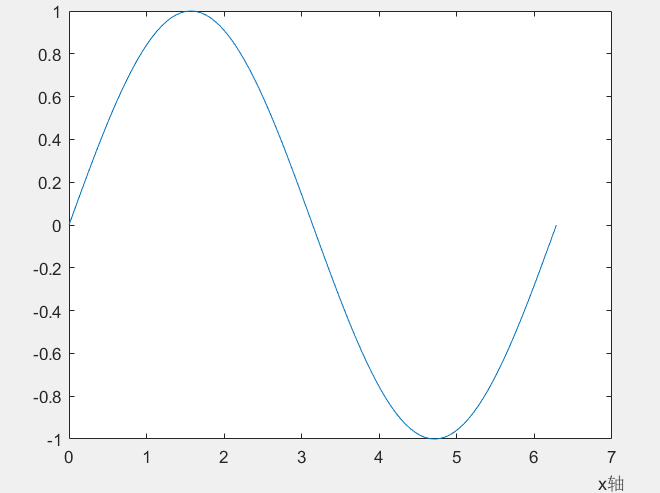参考链接1：http://www.ilovematlab.cn/thread-136589-1-1.html 参考链接2：http://blog.sina.com.cn/s/blog_7e6270010101nt0r.html#commonComment
展开全文• ----------------------------------------------------------------------------------- 运行时间：2.334s运行时间：2.334 xlabel,ylabel 中用法也一样 disp 中用法也一样 （disp：向命令窗口输出）
1个变量：
title ( ['运行时间: ',num2str(toc) ] );

----------------------------------------------------------------------------------- 运行时间：2.334
title ( ['运行时间: ',num2str(toc),'s' ] );

----------------------------------------------------------------------------------- 运行时间：2.334s
2个变量：
title ( ['运行时间: ',num2str(toc),'s','运行时间: ',num2str(toc) ] );

----------------------------------------------------------------------------------- 运行时间：2.334s运行时间：2.334
xlabel,ylabel 中用法也一样
disp 中用法也一样（disp：向命令窗口输出）
展开全文• Matlab作图实例——xlabel,ylabel,title,text,plot,patch,datetime等的应用做线性图，并用变量标记每个点做US inflation相关的图test 绘制区间阴影test 绘制美丽的柱状图新浪微博 做线性图，并用变量标记每个点 ...


Matlab作图实例——xlabel,ylabel,title,text,plot,patch,datetime等的应用
做线性图，并用变量标记每个点画线形函数图，填充一部分并画网格新浪微博

做线性图，并用变量标记每个点

所用数据如下：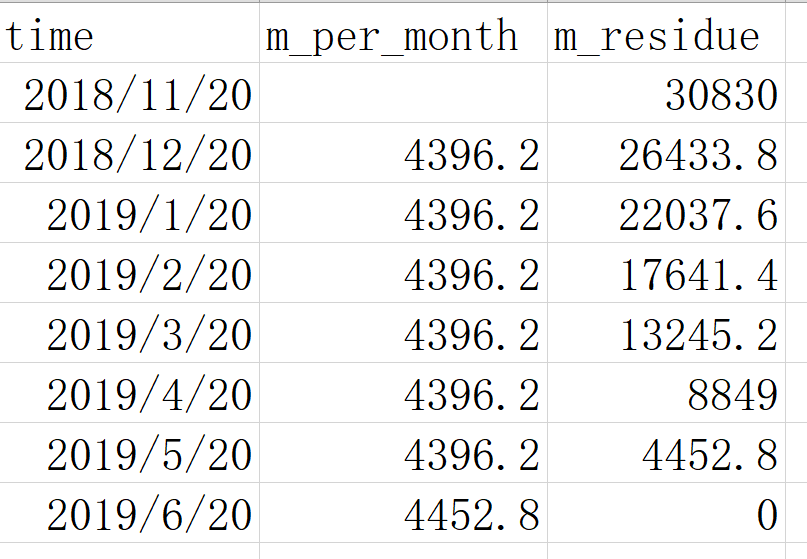代码如下：

clear
clc
format compact
format shortG
T.time=datetime(datestr(T.time,'yyyy.mm.dd'),'InputFormat','yyyy.MM.dd',...
'format','yyyy.MM.dd')
p=plot(T.time,T.m_per_month,T.time,T.m_residue)
p(1).Marker='o'
p(2).Marker='*'
box off
%让y轴不用科学计数法显示
h=gca
y_val=h.YTick
y_str=string(y_val) %等价于y_str=num2str(y_val')
h.YTickLabel=y_str
%横轴日期显示设置
h.XTick=T.time
xtickangle(45) %让x轴的标签逆时针旋转45度
%画垂直虚线
hold on
p1=plot([datetime(2018,11,20) datetime(2018,11,20)],...
[0 30830],'Color',[0.6 0.6 0.6],'LineStyle','--')
p2=plot([datetime(2018,12,20) datetime(2018,12,20)],...
[0 26434],'Color',[0.6 0.6 0.6],'LineStyle','--')
p3=plot([datetime(2019,01,20) datetime(2019,01,20)],...
[0 22038],'Color',[0.6 0.6 0.6],'LineStyle','--')
p4=plot([datetime(2019,02,20) datetime(2019,02,20)],...
[0 17641],'Color',[0.6 0.6 0.6],'LineStyle','--')
p5=plot([datetime(2019,03,20) datetime(2019,03,20)],...
[0 13245],'Color',[0.6 0.6 0.6],'LineStyle','--')
p6=plot([datetime(2019,04,20) datetime(2019,04,20)],...
[0 8849],'Color',[0.6 0.6 0.6],'LineStyle','--')
p7=plot([datetime(2019,05,20) datetime(2019,05,20)],...
[0 4452.8],'Color',[0.6 0.6 0.6],'LineStyle','--')
hold off
%标注每个点
str1=string(T.m_per_month)
str2=string(T.m_residue)
text(T.time,T.m_per_month-1200,str1,'Color',[0 0.447 0.741],...
'HorizontalAlignment','center')
text(datetime(datenum(T.time)+2,'ConvertFrom','datenum'),...
T.m_residue+1100,str2,...
'Color',[0.85 0.325 0.098],...
'HorizontalAlignment','left')
%图例
legend([p(1) p(2)],{'每月还款金额','每月还款后剩余总本息'},...
'Location','northeast','NumColumns',1)
%各个标题
xlabel('还款时间')
ylabel('还款金额')
title({'GGG还款计划';'2018.12.20-2019.06.20'})
print('GGG还款计划','-dpdf')
%将数据再写入excel
% writetable(T,'test.xlsx','WriteVariableNames',true)


做出的图如下：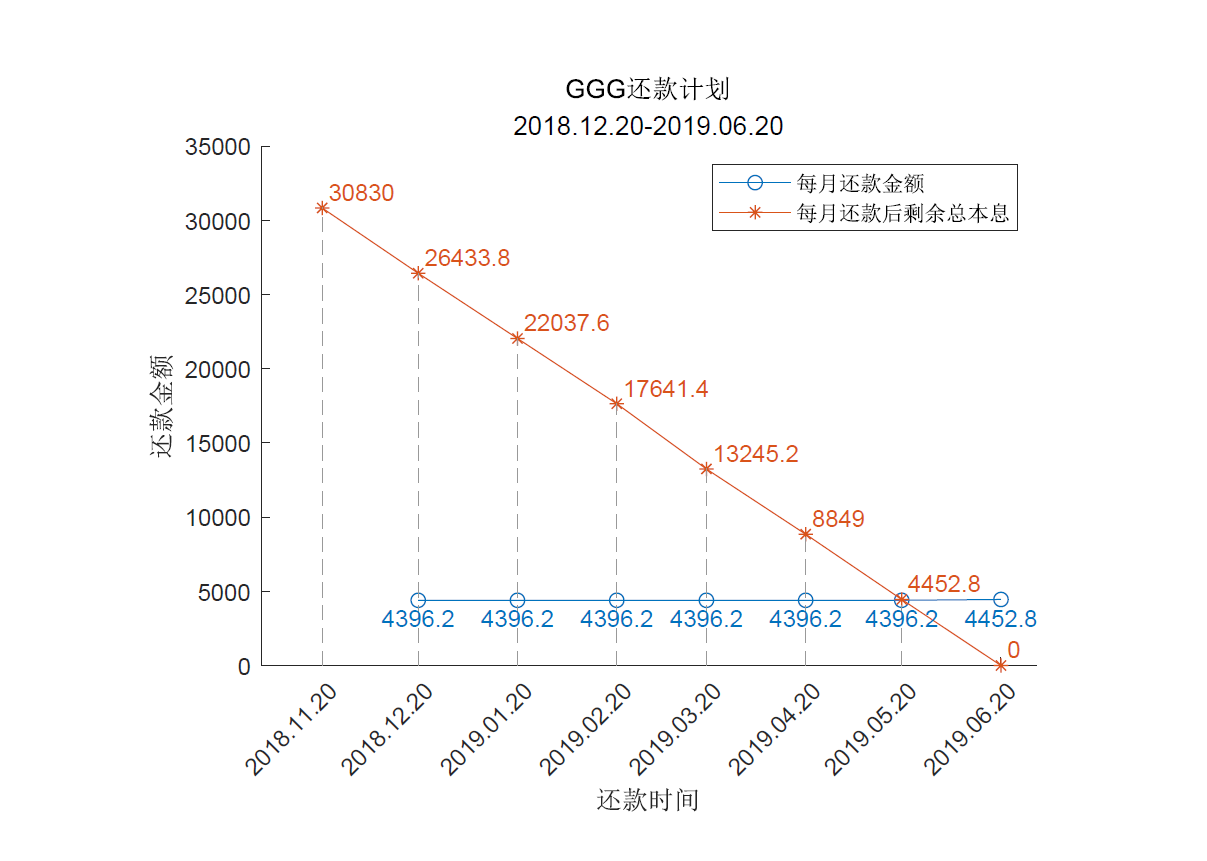画线形函数图，填充一部分并画网格

相应代码为：

%填充并画网格
clear
clc
v1 = [0 0; 4 0; 4 4;0 4];
f1 = [1 2 3 4];
figure
patch('Faces',f1,'Vertices',v1,...
'EdgeColor',[0.75 0.75 0.75],'FaceColor',[0.75 0.75 0.75]);
g=gca
g.XTick=[0:4]
g.YTick=[0:4]
g.XLim=[0 4.5]
g.YLim=[0 4.5]
grid on
g.Layer = 'top';
g.GridColor=[1 1 1]
g.GridLineStyle='--'
g.GridAlpha = 1
axis square
%挖洞
v2 = [1 1;2 1;2 2;1 2];
f2 = [1 2 3 4];
patch('Faces',f2,'Vertices',v2,...
'EdgeColor',[0.75 0.75 0.75],'FaceColor',[1 1 1]);
%画函数图
hold on
f1=@(t) 4*t-4
f2=@(t) 0.25*t+1
f1p=fplot(f1,[1 2],'k','LineWidth',1,'DisplayName','X的策略')
f2p=fplot(f2,[0 4],'--k','LineWidth',1,'DisplayName','Y的策略')
xlabel('X的策略')
ylabel('Y的策略')
legend([f1p f2p],{},'NumColumns',2,'FontSize',10)
%导出为PDF
% saveas(gcf,'qiyan.pdf')
print('qiyan','-dpdf')


做出的图如下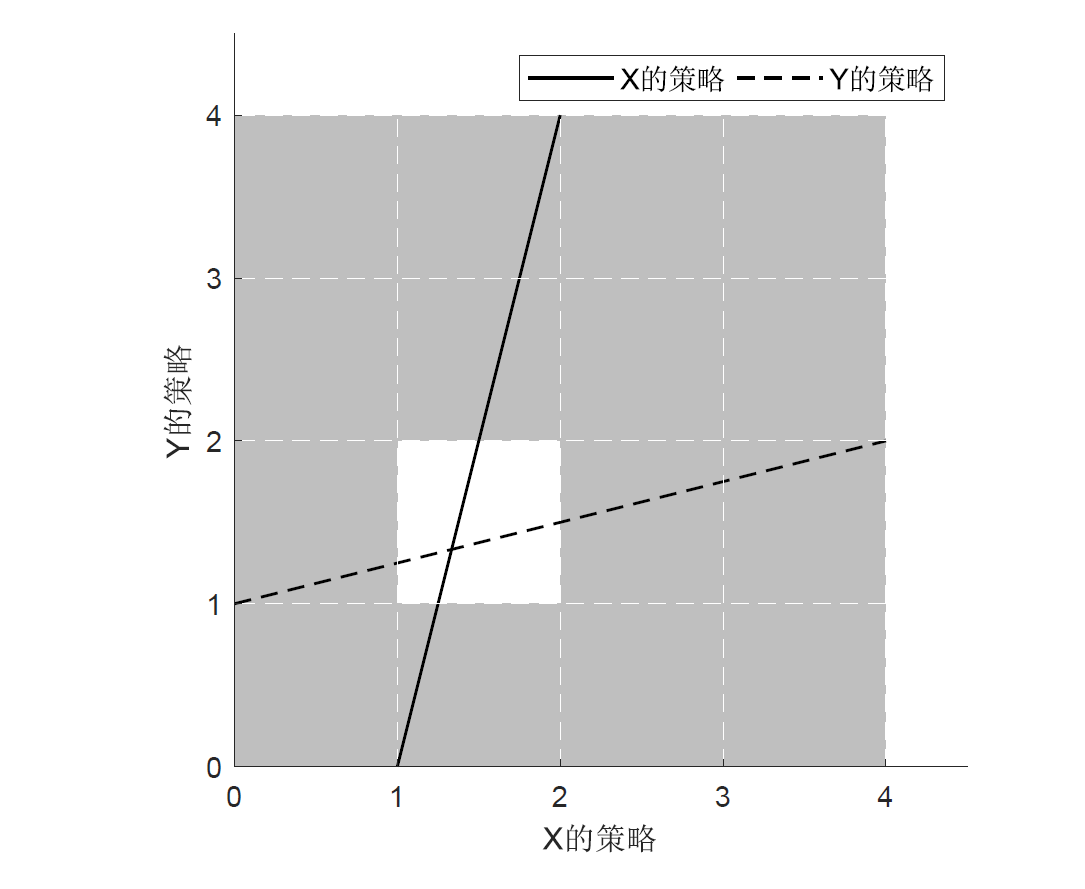新浪微博

可关注我的新浪微博，里边动不动会发一些Stata，Latex，Matlab的tips。当然，本人时不时会背一些英语单词，请不要感到烦躁。

展开全文• 1、问题描述 之前一直使用的都是2015b版本，用了三年也没遇到过这个问题。...查了一圈博客论坛后，问题好像是：不知道从哪个版本开始，matlab的坐标axes默认字体变成了Helvetica，这种字体不支持中文，
1、问题描述
之前一直使用的都是2015b版本，用了三年也没遇到过这个问题。但是最近的某一天，这个著名的问题就出现了。当时打算下载2020a版，就没有太过关注，搜索了一下解决办法，基本都是针对编辑器的默认字体修改，试过了但无效。绘图axes的显示字体好像和编辑器采用的好像不是相同的字体管理方式，但是axes的字体管理界面一直都没有找到。
换了2020a版本之后，仍然呈现方框。查了一圈博客论坛后，问题好像是：不知道从哪个版本开始，matlab的坐标axes默认字体变成了Helvetica，这种字体不支持中文，因此总是会在画图时显示为乱码或方框，我遇到的是方框。
Why is “Helvetica” the default axes font type in MATLAB when this font does not exist on my Windows XP machine?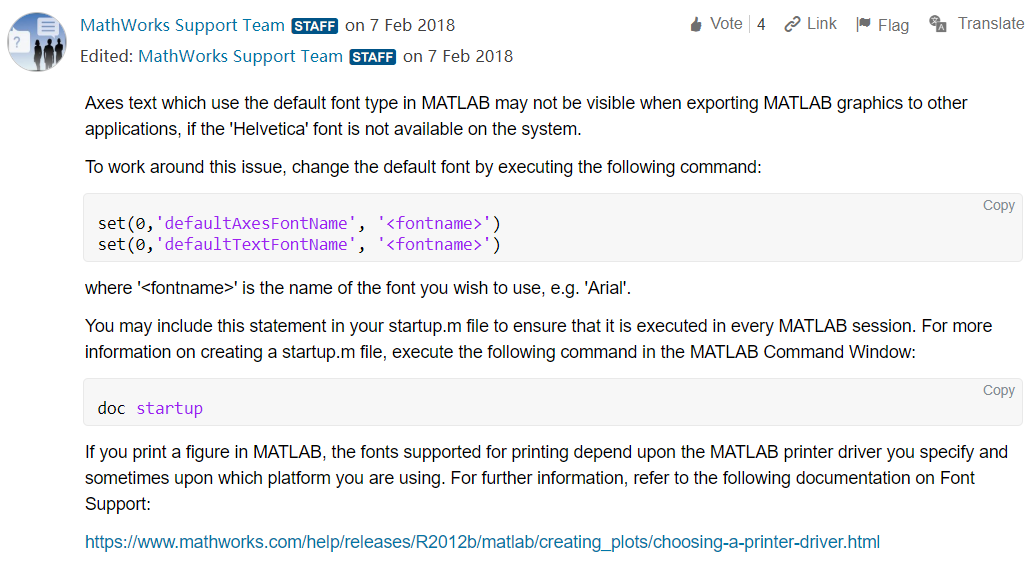matlab技术支持团队的回答只是提供了一种解决方案，但是对于为什么Helvetica是默认字体没有给予解释。
Why does MATLAB use the default Helvetica font when certain True Type fonts are chosen for a text object in my MATLAB figure?
2、问题解决
C = listfonts 	% 可以查看系统中存在的字体
edit listfonts 	% 可以打开系统字体列表文件
Ans = get(0,'defaultAxesFontName');  % 运行结果可看到是Helvetica

总结来看，下面的方法可以解燃眉之急，但也不是一劳永逸，每次打开matlab还是要重复一遍。比较好的方法是在matlab的startup.m文件中加入下面的代码。
set(0,'defaultAxesFontName', 'Monospaced');
set(0,'defaultAxesFontSize', 10);

参考链接： 如何解决 Matlab 画图时中文显示乱码的问题？
展开全文字体 title
• MATLAB 图形标注与修饰函数 在 MATLAB中，可利用 Figure窗口的菜单和工具栏对图形进行标注、修饰等，操作简单，此外，还可以利用 MATLAB中自带的函数来进行图形的修饰。下面将对其进行介绍 绘制图形以后，需要对图形...
• 随时随地阅读更多技术实战干货，获取项目源码、学习资料，请关注源代码社区公众号(ydmsq666)、博主微信（guyun297890152）、QQ技术交流群(183198395)。 标注x，y和z轴 ...xlabel(axes_handl...ylabel 标签
• Matlab图形中特殊字符的标注方法 1首先得到所要标注的图 2在Matlab图形窗口中利用insert->textbox,插入文本窗口在需要标注的位 置 3敲入4个美元符号,如$然后在两两$的中间位置键入反斜杠\ 及所要标注的内容如$\... • 如何解决matlab title, xlabel, legend 中文变成方框的问题 存在的问题 很多小伙伴在使用matlab编写程序的时候，会给结果图加上图标题title(’’)来提醒自己运行结果类别。但是在实际操作中会发现，当图标题为英文时... • ## matlab label倾斜 千次阅读 2020-03-20 19:36:31 在三维坐标系中，由xLabel(‘String’)得到的label是水平处于x轴下方。而三维坐标系中x轴是倾斜的。如何使得到的label处于x轴的tick的下方，且倾斜度与x轴相同？ [x y]=meshgrid(0:0.01:1); z=peaks(101); mesh(x,y,... • pyplot.xlabel(u ' 中文 ' , fontproperties= font_set) 7 pyplot.ylabel(u ' 中文 ' , fontproperties=font_set) 结果 Successfully ！！！ 有趣的事，Python永远不会缺席！还不来加... • Matlab 的 text/ title/ xlabel/ ylabel 函数支持 TeX、LaTeX 排版语法，如希腊字母、上下标、特殊符号等等。 具体流程 调用上述 4 种函数时可以获得相应的句柄，再调用 set 函数向相应句柄设置属性值 txt = xlabel... • xlabel('x','fontsize',20,'fontname','Courier') ylabel('y','fontweight','bold'); 或 set(gca,'String','h(\mum)','color','k','fontsize',12,'fontweight','bold'); 坐标轴加粗 set(line1,'linestyle','-','... • 在一组子图中将文本作为标题，xlabel或ylabel放置。返回标签的句柄和轴的句柄。 [ax，h] = suplabel（text，whichLabel，supAxes） 返回轴和标签的句柄。 ax = suplabel（文字，whichLabel，supAxes） 仅返回轴... • ## MATLAB xlable的使用 千次阅读 2020-09-08 17:33:32 xlabel('正弦函数') 效果： 多行 xlabel({'正弦函数','sin'}) 特殊字符 xlabel({'正弦函数','sin','-2\pi \leq x \leq 2\pi'}) 改变大小及加粗 xlabel({'正弦函数','sin','-2\pi \leq x \leq 2\... • %axis为'x'或'y'，分别表示更改x或y刻度 %ticks是字符cell function settick(axis,ticks) n=length(ticks); tkx=get(gca,'XTick');tky=get(gca,'YTick');... w=linspace(tkx(1),tkx(end),n)... • ## matlab 绘图label字体 千次阅读 2019-05-21 19:05:00 h1=xlabel('$$\rho[1/m^2]$$','FontSize',13); h2=ylabel('v$[m/s]$','FontSize',13); % set(gca,'FontSize',20); set(h1,'Interpreter','latex'); set(h2,'Interpreter','latex') --------------------- 原文：... • ## matlab——修改图中字体 千次阅读 多人点赞 2020-08-29 21:13:32 在画图的时候，我们可以使用xlabel命名x轴的名字，使用ylabel命名y轴的名字，使用legend命名变量的名字，使用title命名图片的标题。但标题的字体，大小都是默认的。其实那些都是可以调整的，这篇文章就介绍下，... • ## matlab XTickLabel 旋转 千次阅读 2017-11-27 16:55:13 set(gca,’XTickLabel’,{‘农作物成灾面积’,’地震灾害损失’,’海洋灾害损失’,’森林火灾损失’,’地质灾害损失’}) xtb = get(gca,’XTickLabel’...xlabel(‘时间’),ylabel(‘关联度’) %设置x轴和y轴的名称 • 搜了一圈发现MATLAB并没有实现这个功能的函数，于是只能使用text行数进行实现。实现过程主要是先调整gca的宽度，然后上移为两行text腾出空间。然后设置gca本身的xticklabels为空。根据xticks的位置和y轴的范围设置两... • 可以在matlab 中画图设置横坐标字体为斜体，美观 • 同一个坐标轴上的两个字母 X 和 m， 希望X 是斜体，m是...在MATLAB中，该如何实现X 是斜体，m是正体。解决以上问题：figure, xlabel('\itX, \rmm')那么，如果要设置xlabel('\itX, \rmm')里面的x,m的字体为Times ... • ## Matlab设置字体大小 万次阅读 2018-09-08 09:52:02 x轴的名称及字体和大小：xlabel('x(m)','FontName','Times New Roman','FontSize',7); y轴的名称及字体和大小：ylabel('zc(m) and y(m)','FontName','Times New Roman','FontSize',7,LineWidth',1.5); ... • 最近用matlab绘制折线图，有一些常用的指令记录下来。 首先是加速比的程序代码，具体功能记录在注释里面。 x1 = [8 16 32 64 128 256]; x2 = [32 64 128 256]; y = [1 2 4 8 16 32]; y04 = [1 1.7872 3.3846 6....文字加粗 • x=0:0.25:2.25;...h1=xlabel('$$\rho[1/m^2]$$','FontSize',13); h2=ylabel('v$[m/s]\$','FontSize',13); % set(gca,'FontSize',20); set(h1,'Interpreter','latex'); set(h2,'Interpreter','latex')
• 在 ubuntu 14.04 中，使用 Matlab 2012a plot，发现 title 的 font 无法修改，也无法修改大小， sudo apt-get install xfonts-base xfonts-100dpi xfonts-75dpi gsfonts-X11 gsfonts-other 如果要检查...
• MATLAB中可以使用的一些命令，在坐标轴中可以显示希腊字母。 但是有些字母可能找不到，或者MATLAB已经不支持，如\varepsilon，所以就需要使用字符编码来实现 一些特殊字符的编码如下： >> char([900:1000])...
• ## MATLAB——阈值分割（一）

万次阅读 多人点赞 2018-11-01 23:44:51
xlabel('(b)迭代法全局阈值分割'); Th=graythresh(f);%阈值 display('Global Thresholding- Otsu''s Method'); Th s=im2bw(f,Th); subplot(223);imshow(s); xlabel('(c)全局阈值Otsu法阈值分割'); se=strel('disk',...图像处理 阈值分割 直方图分割...

# matlab的xlabelmatlab 订阅